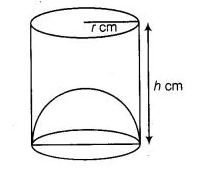# The capacity of a cylindrical vessel with a hemispherical

Question:

The capacity of a cylindrical vessel with a hemispherical portion raised upward at the bottom as shown in the figure is $\frac{\pi r^{2}}{3}[3 h-2 r]$.Solution:

True

We know that, capacity of cylindrical vessel = πr2h cm3

and capacity of hemisphere $=\frac{2}{3} \pi r^{3} \mathrm{~cm}$

From the figure, capacity of the cylindrical vessel

$=\pi r^{2} h-\frac{2}{3} \pi r^{3}=\frac{1}{3} \pi r^{2}[3 h-2 r]$